# Thread: Doubly Related Rates problem

1. ## Doubly Related Rates problem

Could someone please help me figure this problem out?

A company, producing muesli bars, faces the demand equation x3+xp+p3=143 between price and quantity, where the quantity x is measured in millions. The cost to produce 1 million units increase over time according to the function k(t)=200t. We are presently in time t=25. The company has achieved a constant increase of the profit of 1000000 dollars per year and expects to maintain this standard.

a) Presently, 5000000 units are produced and sold at a price of 2dollars per piece. At what rate is the production level x increasing or decreasing? What about the rate of change for the price?

b) More generally, try to express the rate of change of x (over time) in terms of x and p. Can you express it in terms of x alone? Can you express it in terms of time t? If not, try to describe why not.

c) Can you explain the difference of the setting of this exercise and the ordinary related rates situation?

2. I may be way off here, but...

we have profit = revenue minus cost, i.e.

s = r - k

and revenue = quantity sold times price, ie.

r = 1,000,000 xp

and IF (but here's where I wouldn't like to bet) we can say that the cost to produce x million units increases over time according to the function

k(x,t) = 200 xt

... then (and only if a picture helps, pardon if not) we haveFull-size:

Spoiler: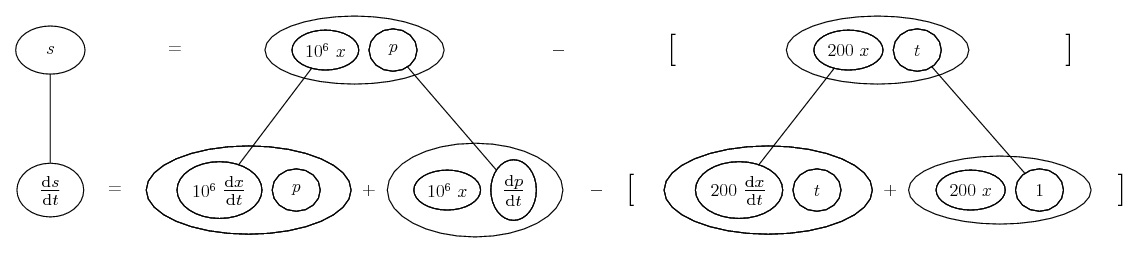... where... is the product rule. Straight lines differentiate downwards with respect to t.

Also, we can use...... the chain rule, to express $\displaystyle \frac{\mathrm{d}p}{\mathrm{d}t}$ as the product of $\displaystyle \frac{\mathrm{d}p}{\mathrm{d}x}$ and $\displaystyle \frac{\mathrm{d}x}{\mathrm{d}t}$ i.e...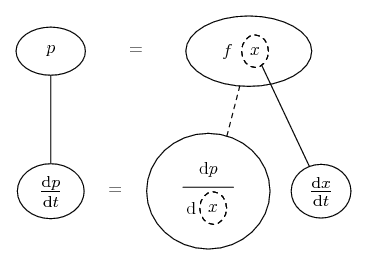Straight continuous lines still differentiate downwards with respect to t, and the straight dashed line similarly but with respect to the dashed balloon expression (which is the inner function of the composite and hence subject to the chain rule).

And we can find $\displaystyle \frac{\mathrm{d}p}{\mathrm{d}x}$ by implicitly differentiating the demand formula...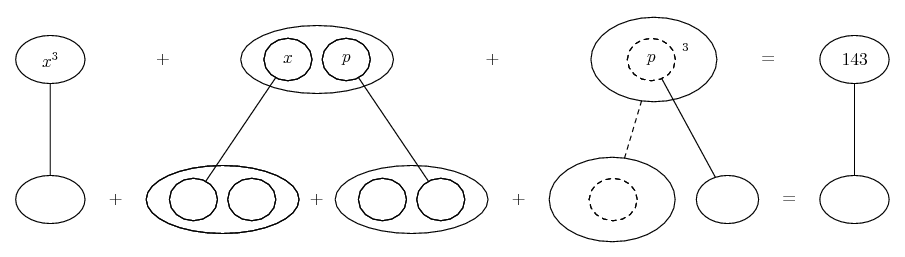Spoiler: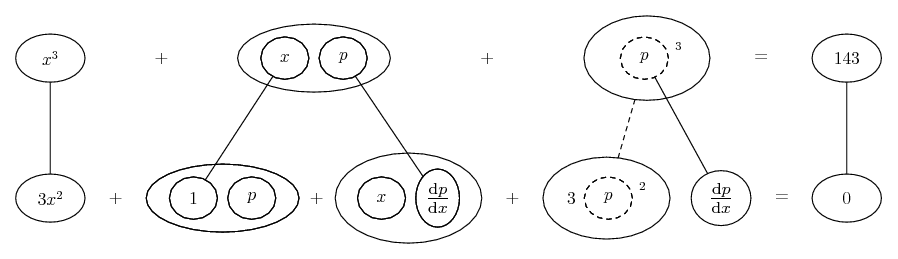Solving the bottom row gives...

$\displaystyle \frac{\mathrm{d}p}{\mathrm{d}x} = -\ \frac{3x^2 + p}{3p^2 + x}$

Plugging in...

Spoiler: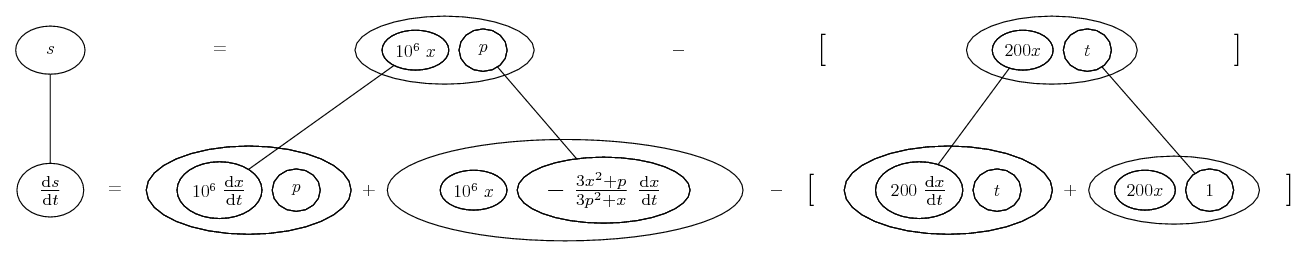Spoiler: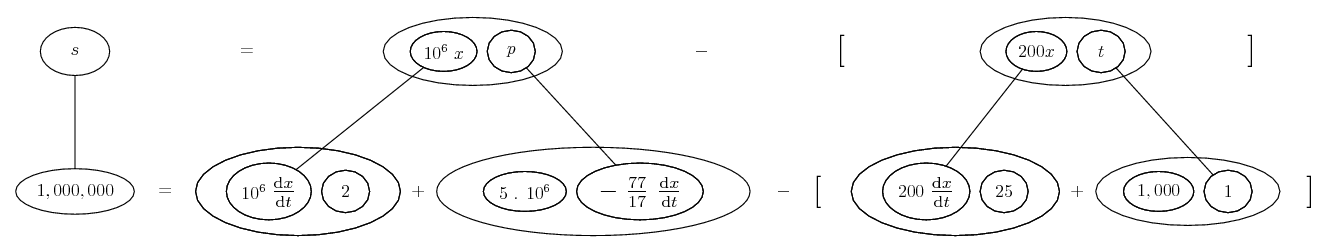Maybe solving the bottom row there will help with a and some of b (though quite possibly in the wrong order).

__________________________________________
Don't integrate - balloontegrate!

Balloon Calculus; standard integrals, derivatives and methods

Balloon Calculus Drawing with LaTeX and Asymptote!

#### Search Tags

doubly, problem, rates, related